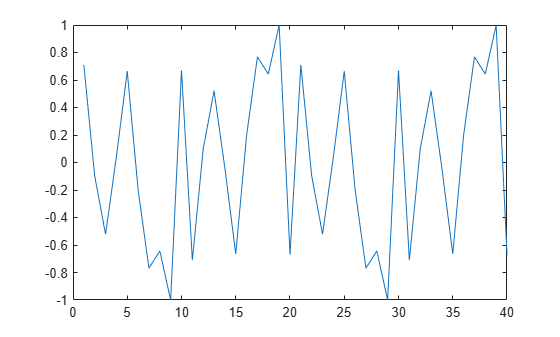Documentation

resample

Resample time-domain data by decimation or interpolation (requires Signal Processing Toolbox software)

Syntax

resample(data,P,Q)
resample(data,P,Q,order)

Description

resample(data,P,Q) resamples data such that the data is interpolated by a factor P and then decimated by a factor Q. resample(z,1,Q) results in decimation by a factor Q.

resample(data,P,Q,order) filters the data by applying a filter of specified order before interpolation and decimation.

Input Arguments

data

Name of time-domain iddata object. Can be input-output or time-series data.

Data must be sampled at equal time intervals.

P, Q

Integers that specify the resampling factor, such that the new sample time is Q/P times the original one.

(Q/P)>1 results in decimation and (Q/P)<1 results in interpolation.

order

Order of the filters applied before interpolation and decimation.

Default: 10

Examples

collapse all

Increase the sampling rate of data by a factor of 1.5 and compare the resampled and the original data signals.

u = idinput([20 1 2],'sine',[],[],[5 10 1]);
u = iddata([],u,1);
plot(u)
ur = resample(u,3,2);
plot(u,ur)Algorithms

If you have installed the Signal Processing Toolbox™ software, resample calls the Signal Processing Toolbox resample function. The algorithm takes into account the intersample characteristics of the input signal, as described by data.InterSample.